Hello everyone,

Currently i’m trying to implement an attention mechanism for video representation but i’m having vanishing gradient issues in the training process, I have been debugging for hours but I still can’t find the problem with my code, that’s why I’m asking for help if it has is any error that could be causing this problem. I’ll explain the mechanism, the paper of reference can be found here.

Letbe a set of embedding vectors of a video with N frames. They are extracted using a pretrained convolutional network like Resnet152 or Resnext101. Then, to extract temporal information, they are feed as “word embedding” into a GRU to get a set of N hidden states.

With this, they createto extract spatial and temporal information of the video.

Then they use an attention mechanism to get a single vector representation for the video. To get the attention mapthey use the mean of thevectors, called.

They calculate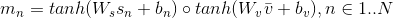(1)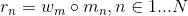(2)(3)

where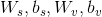are trainable weights and bias,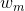is a trainable vector andis the element-wise product operation.

Then, to get the single vector representation of the setthey calculate:(4)

Now that i explained the mechanism I’m trying to implement, the following code is what I’ve wrote:

``````class LinearVector(nn.Module):
# this module is for the trainable vector W_m
def __init__(self, size):
super(LinearVector, self).__init__()
stdw = 1. / math.sqrt(self.weight.size(0))
self.weight.data.normal_(mean=0, std=stdw)

def forward(self, m):
return self.weight * m

class VideoBranch(nn.Module):

def __init__(self, resnet152_dim, gru_dim, att_dim, out_dim, device):  # 2048, 1024, 1024, 2048, gpu:0

super(VideoBranch, self).__init__()
self.device = device
self.gru = nn.GRU(resnet152_dim, gru_dim, batch_first=True)

self.w_v = nn.Linear(resnet152_dim, att_dim)
self.w_s = nn.Linear(resnet152_dim + gru_dim, att_dim)
self.w_m = LinearVector(att_dim)

self.dropout_1 = nn.Dropout(0.2)
self.dense_1 = nn.Linear(resnet152_dim + gru_dim, out_dim, bias=False)
self.dense_1_bn = nn.BatchNorm1d(out_dim)
self.dropout_2 = nn.Dropout(0.2)
self.dense_2 = nn.Linear(out_dim, out_dim, bias=False)
self.dense_2_bn = nn.BatchNorm1d(out_dim)

def forward(self, resnet152_vectors, n_frames):  #B = batch size, N = max length sequence
batch_size = resnet152_vectors.size(0)
N = n_frames.max().item()
resnet152_vectors = resnet152_vectors[:, :N, :] # delete unnecesary padding.  size = [B, N, 2048]
embs = pack_padded_sequence(resnet152_vectors, n_frames, batch_first=True, enforce_sorted=False)
h_packed, _ = self.gru(embs)
h, _ = pad_packed_sequence(h_packed, batch_first=True) # size of h = [B, N, 1024]

# attention mechanism
s = torch.cat((h, resnet152_vectors), 2).view(batch_size, N, -1) # size = [B, N, 1024 + 2048]
v = resnet152_vectors.sum(dim=1) / n_frames.view(batch_size, -1).float()  # resnet152_vectors.mean() ignoring padding. size = [B, 2048]
y_s = self.w_s(s).tanh()  # size = [B, N, 1024] 1024 is the att_size
y_v = self.w_v(v).tanh()  # size = [B, 1024]
m = torch.mul(y_s, y_v.view(batch_size, 1, -1)) # equation (1), size=[B, N, 1024]

# from here, the gradient vanishes  for W_s, W_v and the GRU

r = self.w_m(m)  # equation (2), size=[B, N, 1024]
alpha = torch.sum(r, dim=2).softmax(dim=1) # equation (3), size = [B, N]
alpha = alpha.view(batch_size, N, 1)   # size = [B, N, 1]
v_a = torch.sum(torch.mul(alpha, s), dim=1) # equation (4), size = [B, 2048]

# fully-connected mapping to another vector space
y = self.dense_1(v_a)
y = self.dense_1_bn(y)
y = nn.ReLU()(y)
y = self.dropout_1(y)
y = self.dense_2(y)
y = self.dense_2_bn(y)
y = nn.ReLU()(y)
y = self.dropout_2(y)
return y
``````

Using a gradient flow plot shared by RoshanRane, at the start of the training process the gradient starts to vanish for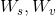and the GRU.and after a few batches the gradient completely vanishIs there any error in this implementation? Help would be very appreciated.
Thank you very much!